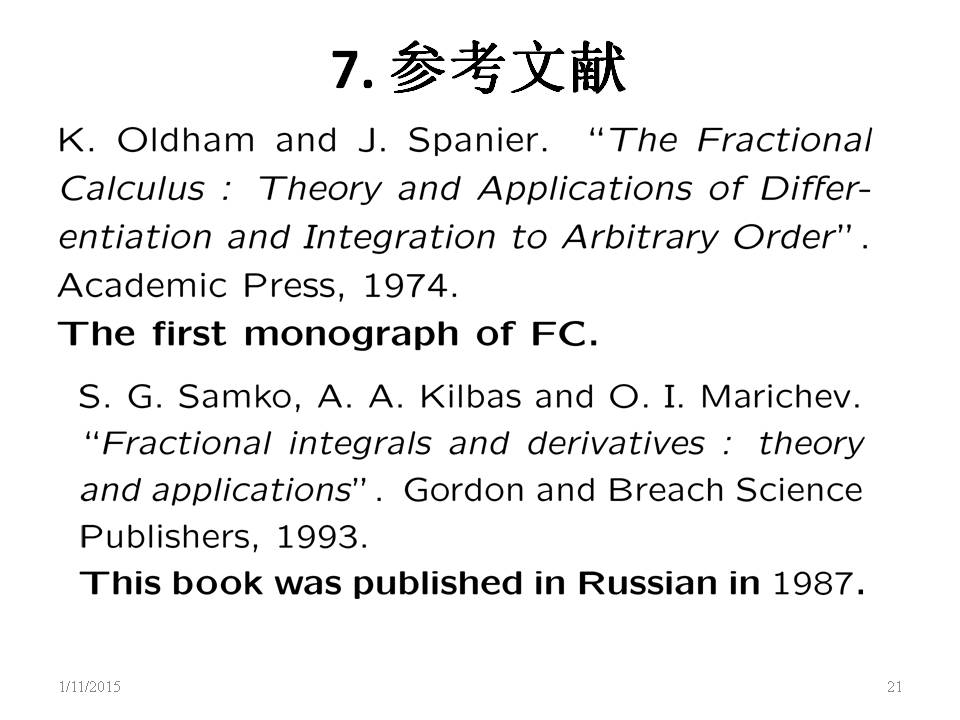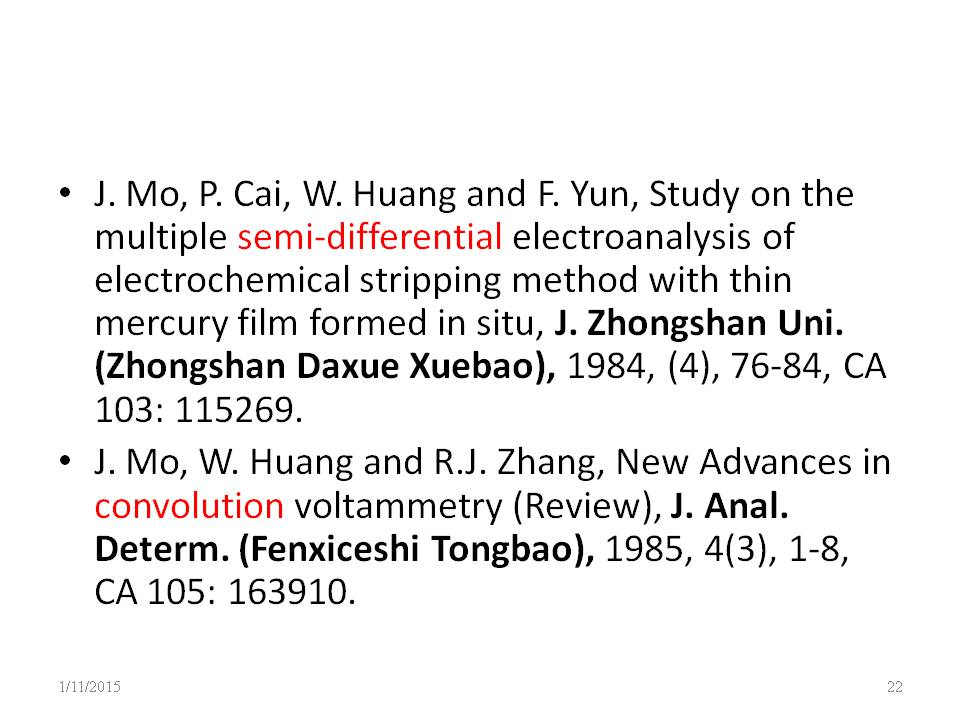﻿ ﻿﻿﻿ Fractional Calculus Computer Algebra System math software++=﻿

# Fractional Differential Equation

1. ## Introduction

The science of physics is built fundamentally upon differential equations. Many of the most useful differential equations appearing in physics are of partial differential equations, known as math physical equations, so that understanding the solutions that can arise and their interconnections has much to say about possible systems amenable to analyze physical problems.

In mathematics, a partial differential equation (PDE) is a differential equation that contains unknown multivariable functions and their partial derivatives. PDEs are used to formulate problems involving functions of several variables, and are either solved by computers, or used to create a computer model. A special case is ordinary differential equations (ODEs), which deal with functions of a single variable and their derivatives.

PDEs can be used to describe a wide variety of phenomena such as sound, heat, diffusion, electrostatics, electrodynamics, fluid dynamics, elasticity, gravitation and quantum mechanics. These seemingly distinct physical phenomena can be formalised similarly in terms of PDEs. Just as ordinary differential equations often model one-dimensional dynamical systems, partial differential equations often model multidimensional systems.

Fractional differential equations can describe the dynamics of several complex and nonlocal systems with memory. They arise in many scientific and engineering areas such as physics, chemistry, biology, biophysics, economics, control theory, signal and image processing, etc. Particularly, nonlinear systems describing different phenomena can be modeled with fractional derivatives. Chaotic behavior has also been reported in some fractional models. There exist theoretical results related to existence and uniqueness of solutions to initial and boundary value problems with fractional differential equations.

2. ## Differential equation

By default, the unknown function is y(x) and its initial value is y(0). An independent variable is x for ordinary differential equation. For a partial differential equation, the unknown function is y(t,x) and its two independent variables are x and t. For a system of equations, two unknown functions are x(t) and y(t) with an independent variable t.

By default, the Caputo definition of fractional calculus is used by dsolve(). If you want to use the Riemann defintion, use the Laplace transform solver lasolve(). Difference between Caputo definition and the Riemann-Liouville (R-L) definition are in section 6 of fractional calculus

lasolve(y(-0.5,x)=1) give nonzero.
dsolve(y(-0.5,x)=1) give zero.

there are 4 way to input derivative of y: y', y(1,x), ds(y,x), d(y(x))
there are 3 way to input second order derivative of y: y(2,x), ds(y,x,2), d(y(x),x,2)
there are 3 way to input the 0.5 order derivativeof y: y(0.5,x), ds(y,x,0.5), d(y(x),x,0.5)
there are 4 way to input the 0.5 order integral of y: ints(y,x,0.5), y(-0.5,x), ds(y,x,-0.5), d(y(x),x,-0.5)

Input y(1,x) - 2y = 0 as first order differential equation
dy/dx - 2y=0
Input your equation into mathHand.com, click the "dsolve" button for solution, then click the "test" button to test its solution.

### Solve graphically

Some differential equations cannot be solved symbolly, but can be solved graphically by a plot function odeplot( ), then tick the y'= or y"= checkbox. e.g.
y' = sin(x)-cos(y)

3. ## Partial differential equation

Input your equation, click the dsolve button, then click the plot2D button to show its curve graph where you can change a time t value, or click the plot3D button to show its 3D graph where you can spin graph..

### A first order differential time equation

Input ds(y,t,1) = ds(y,x,2)-ds(y,x)-cos(x)-exp(t) as a first order partial differential time t in the left hand side of the equation,
(dy)/dt = (d^2y)/dx^2-dy/dx-exp(t)-exp(x)
e.g. a diffusion equation:
(dy)/dt = (d^2y)/dx^2 - dy/dx = 0
e.g. a diffusion equation in 2 dimemtions:
(dy)/dt - (d^2y)/dx^2 - (d^2y)/(du^2) = 0
e.g. a spherical diffusion equation:
(dy)/dt - (d^2y)/(dx^2)-1/x*dy/(dx)-y =0
e.g. a diffusion equation in 3 dimemtions:
(dy)/(dt) - (d^2y)/dx^2 - (d^2y)/(du^2) - (d^2y)/(dv^2) = 0

### A second order differential time equation,

Input ds(y,t,2) = ds(y,x,2)-ds(y,x)-cos(x)-exp(t) as second order partial differential time t in the left hand side of the equation,
(d^2y)/dt^2 = (d^2y)/dx^2-dy/dx-exp(t)-exp(x)
e.g. a wave equation:
(d^2y)/dt^2 = (d^2y)/dx^2-dy/dx-cos(x)
e.g. a wave equation in 2 dimemtions:
(d^2y)/(dt^2) - (d^2y)/dx^2 - (d^2y)/(du^2) = 0
e.g. a wave equation in 3 dimemtions:
(d^2y)/(dt^2) - (d^2y)/dx^2 - (d^2y)/(du^2) - (d^2y)/(dv^2) = 0

4. ## Integral equation

Integral equation is equation with integral. e.g.
Intput integrates(y) = 2y for int y dx = 2y
Input ds(y,x, -1) = 2y for d^-1/dx^-1 y = 2y

Integral equation can be converted to differential equation by differentiating both sides, but not every integral equation can.

5. ## Double Integral equation

Integral equation is equation with integral. e.g.
Intput ds(y,x,-2) = 2y for int int y (dx)^2 = 2y

6. ## Fractional differential equation

Differential and integral equations can be extend to fractional differential and integral equations by fractional order of fractional calculus.
Input ds(y,x,0.5) = 2y as semi differential equation
d^0.5/dx^0.5 y = 2y

Property of a fractional differential equation is the same as a differential equation.
Solution of linear fractional differential equation = general solution + parcular solution = gsolution()+psolution(),
similar to linear differential equation. So method to solve fractional differential equation is similar to differential equation.

Solve (fractional) differential equation for y by dsolve() or lasolve(), e.g.
dsolve( y'=2y )
dsolve( d^0.5/dx^0.5 y=2y )
lasolve( d^0.5/dx^0.5 y=2y )

7. ## Fractional partial differential equation

Consider a time fractional partial differential equation with fractional order differential time.
Input ds(y,t,0.5)=ds(y,x,2)-ds(y,x)-cos(x)-exp(t) as semi partial differential time t in the left hand side of the equation,
(d^0.5y)/dt^0.5 = (d^2y)/dx^2-dy/dx-cos(x)-exp(t)
(d^0.5y)/dt^0.5 = (d^2y)/dx^2-dy/dx-exp(t)-exp(x)

8. ## Fractional integral equation

Integral equation is extend to fractional integral equation by minus fractional order.
Intput integrates(y,x,0.5) = 2y for int y (dx)^0.5 = 2y
Input ds(y,x, -0.5) = 2y as semi integral equation for
d^-0.5/dx^-0.5 y = 2y

Integral equation can be converted to differential equation by differentiating both sides. Some fractional integral equation aslo can be converted to fractional differential equation, but not every fractional integral equation can.

9. ## Equation of mixed Fractional differential and integral orders

A mix of differential and integral equation, e.g.
d^(pi)/dx^(pi) y - int y(x) (dx)^pi = 2exp(x)

10. ## System of Fractional differential equations

A system of differential equations is extend to system of fractional differential equation by fractional order. e.g.
(d^0.5x)/dt^0.5=2x, (d^0.5y)/dt^0.5=x+y

By default, the unknown function are x(t) and y(t) and their variable is t, their initial value are x(0) and y(0).
dsolve(x(1,t)=x-t,y(1,t)=t-x)
x(0):=1, y(0):=1, lasolve(x(1,t)=x-t,y(1,t)=t-x)

11. ## Complex order differential equation

Differential equation is extend to complex order differential equation by extension of order to complex number.
Input ds(y,x,i) - 2y -exp(x) =0 as complex order differential equation
d^(i)/dx^(i) y - 2y - exp(x)=0

12. ## Variable order differential equation

When the order is variable, it is a variable order differential equation. e.g. the order is changing from -1 to 1 in the sin(x) order differential equation.
(d^sin(x) y)/dx^sin(x)-2y-exp(x)=0
(d^cos(x) y)/dx^cos(x)-4y-exp(x)=0

The order change is similar to a change of the n-order fractional derivative d^n/dx^n x in below picture.The order changes from -1 to 1.

13. ## Test solution

Test solution for (fractional) differential equation by the solutions back into equation to check if result is zero. There sre three ways to check solutions:
1. Click the "test" button to test solution if it is 0, then click the plot2D button to show a line of 0.
2. Use the test() to test solution by test(solution, equation), e.g.
test( exp(4x), d^0.5/dx^0.5 y=2y )
f:= 2y+1, eq:=y'-f=0, s:=dsolve(eq), test(s, eq)
f:= 2y+1, eq:=ds(y,x,0.5)-f=0, s:=dsolve(eq), test(s, eq)
3. the ntest() is numeric test to put a random number into independent variable back to the equation by ntest(solution, equation).

14. ## Comparison of differential equations

Ordernameequationgeneral solutionparcular solution
icomplex order differential equation d^i/dx^i y - 2y=exp(x)C_1*exp(1/2^i*x)-exp(x)
2second order differential equation d^2/dx^2 y - 2y=exp(x)C_1*exp(sqrt(2)*x)-exp(x)
1.51.5 order differential equation d^1.5/dx^1.5 y - 2y=exp(x)C_1*exp(2^(2/3)*x)-exp(x)
1 differential equation d/dx y -2y=exp(x)C_1*exp(2*x)-exp(x)
0.5semi differential equation d^0.5/dx^0.5 y - 2y=exp(x)C_1*exp(4*x)-exp(x)
-0.5semi integral equation d^-0.5/dx^-0.5 y - 2y=exp(x)C_1*exp(1/4*x)-exp(x)
-1integral equation int y\ dx-2y=exp(x)C_1*exp(1/2*x)-exp(x)
solution= general solution+parcular solution = gsolution()+psolution()

All of their solutions are in the same format exp(k x). When the n-order differential equation decreased from 2 to 0.5, its general solution increased from exp(sqrt(2)*x) to exp(4x). When the n-order differential equation decreased from -0.5 to -1, its general solution increased from exp(1/4 x) to exp(1/2 x). but their parcular solutions are unchanged.

15. ## What MathHand can do while other software cannot

MathHand can solve equations which other software cannot, e.g. Wolfram Bugs
16. ## References1. math handbook: chapter 13
2. Roubíček, T. (2013), Nonlinear Partial Differential Equations with Applications (2nd ed.), Basel, Boston, Berlin: Birkhäuser, doi:10.1007/978-3-0348-0513-1, ISBN 978-3-0348-0512-4, MR 3014456.
3. Samko, S. G. (1987), Fractional Integrals And Derivatives - Theory and Applications, 1987.
4. Loverro, Adam (2004), Fractional Calculus - History, Definitions, and Applications for the Engineer, 2004.
5. Baleanu, D. and Kumar, D. (2019), fractional calculus and its applications in physics. 2019.
﻿
See Also